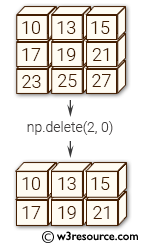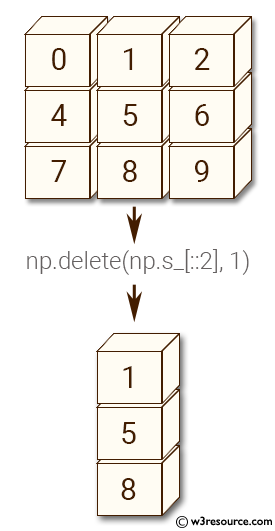# NumPy: delete() function

## numpy.delete() function

The delete() function returns a new array with sub-arrays along an axis deleted. For a one dimensional array, this returns those entries not returned by arr[obj].

Syntax:

`numpy.delete(arr, obj, axis=None)`Version: 1.15.0

Parameter:

Name Description Required /
Optional
arr Input array. Required
obj Indicate which sub-arrays to remove. Required
axis The axis along which to delete the subarray defined by obj. If axis is None, obj is applied to the flattened array. Optional

Return value:

[ndarray] A copy of arr with the elements specified by obj removed. Note that delete does not occur in-place. If axis is None, out is a flattened array.

Example-1: numpy.delete()

``````>>> import numpy as np
>>> arr = np.array([[0,1,2], [4,5,6], [7,8,9]])
>>> arr
array([[0, 1, 2],
[4, 5, 6],
[7, 8, 9]])
>>> np.delete(arr, 1, 0)
array([[0, 1, 2],
[7, 8, 9]])
``````

Pictorial Presentation:Example-2: numpy.delete()

``````>>> import numpy as np
>>> np.delete(arr, np.s_[::2], 1)
array([,
,
])
>>> np.delete(arr, [1, 2, 5], None)
array([0, 4, 5, 7, 8, 9])
``````

Pictorial Presentation:Python - NumPy Code Editor:

Previous: repeat()
Next: insert()

﻿